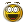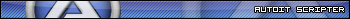Recommended Posts

...without using the "send" command.

Once complete-gets the result from the calculator display and show it in a message box (this wont be the difficult part).  I just need it to simulate the clicks to add two numbers, but I've hit a total mental block and I'm fairly new to AutoIT.  I just commented out the very end where I got stuck so please excuse the sloppiness lol.  At that point I was just writing in things to try and refresh any memory I have of coding. Thanks a ton in advance for any assistance..

While 1
; Asks user for first number in the adding equation

Local \$iNum1 = InputBox('Adding!', 'Enter your first number')

; Validates input is a positive integer
If \$iNum1 < 0 Then
MsgBox(0, 'Error', 'Please enter a valid positive integer')
Else
ExitLoop
EndIf
WEnd

While 2
; Asks user for second number in the adding equation

Local \$iNum2 = InputBox('Adding!', 'Enter your second number')

; Validates input is a positive integer
If \$iNum2 < 0 Then
MsgBox(0, 'Error', 'Please enter a valid positive integer')
Else
ExitLoop
EndIf
WEnd

; Calculates the two inputs without using "send" command

Run('calc.exe', @SystemDir)
WinWaitActive('Calculator')

ControlClick('Calculator', '', '130')
ControlClick('Calculator', '', '131')
ControlClick('Calculator', '', '132')
ControlClick('Calculator', '', '133')
ControlClick('Calculator', '', '134')
ControlClick('Calculator', '', '135')
ControlClick('Calculator', '', '136')
ControlClick('Calculator', '', '137')
ControlClick('Calculator', '', '138')
ControlClick('Calculator', '', '139')

#cs

\$x = \$iNum1

\$z = StringLen(\$x)

For \$iNum1 = 0 to \$z
Case 1

Share on other sites

You are close.  The control ID numbers should be sent as numbers though, not strings (in other words, do not quote them like that).

See if this makes sense

While 1
; Asks user for first number in the adding equation

Local \$iNum1 = InputBox('Adding!', 'Enter your first number')

; Validates input is a positive integer
If \$iNum1 < 0 Then
MsgBox(0, 'Error', 'Please enter a valid positive integer')
Else
ExitLoop
EndIf
WEnd

While 2
; Asks user for second number in the adding equation

Local \$iNum2 = InputBox('Adding!', 'Enter your second number')

; Validates input is a positive integer
If \$iNum2 < 0 Then
MsgBox(0, 'Error', 'Please enter a valid positive integer')
Else
ExitLoop
EndIf
WEnd

; Calculates the two inputs without using "send" command

Run('calc.exe', @SystemDir)
WinWaitActive('Calculator')

;Storing the numbers 0-9 in this array
Local \$aCalcNum = [130,131,132,133,134,135,136,137,138,139]

;Storing control ID for plus and equals
Local \$iCalcPlus = 93, \$iCalcEq = 121

;Enter the first number
For \$i = 1 To StringLen(\$iNum1)
ControlClick('Calculator','', \$aCalcNum[StringMid(\$iNum1, \$i, 1)])
Next

;Hit plus
ControlClick('Calculator','', \$iCalcPlus)

;Enter the second number
For \$i = 1 To StringLen(\$iNum2)
ControlClick('Calculator','', \$aCalcNum[StringMid(\$iNum2, \$i, 1)])
Next

;Hit equals
ControlClick('Calculator','', \$iCalcEq)

;Get visible text from calc window and put in msgbox

Share on other sites

Can you please tell us why you need to use calc to calculate the result?

My UDFs and Tutorials:

Spoiler

UDFs:
Active Directory (NEW 2019-08-19 - Version 1.4.13.0) - Download - General Help & Support - Example Scripts - Wiki
OutlookEX (NEW 2019-07-24 - Version 1.3.6.0) - Download - General Help & Support - Example Scripts - Wiki
Outlook Tools (NEW 2019-07-22 - Version 0.6.0.0) - Download - General Help & Support - Wiki
ExcelChart (2017-07-21 - Version 0.4.0.1) - Download - General Help & Support - Example Scripts
PowerPoint (2017-06-06 - Version 0.0.5.0) - Download - General Help & Support
Excel - Example Scripts - Wiki
Word - Wiki
Taks Scheduler (NEW 2019-10-09 - Version 0.9.0.0) - Download - General Help & Support - Wiki

Tutorials:

Share on other sites

Maybe something like this:

WinWaitActive("[CLASS:CalcFrame]")
ControlClick("[CLASS:CalcFrame]", '', 135)
ControlClick("[CLASS:CalcFrame]", '', 93)
ControlClick("[CLASS:CalcFrame]", '', 135)
ControlClick("[CLASS:CalcFrame]", '', 121)
\$text = WinGetText("[CLASS:CalcFrame]", "")
MsgBox(0, "Texto leído:", \$text)

I prefer use autoit for maths

regardsDanysys.comAutoIt...

Share on other sites

Can you please tell us why you need to use calc to calculate the result?

I prefer use autoit for maths

I guess I was running off the assumption that this was just for the OPs education.  Building my own calculator was actually one of the first scripts that I wrote in autoit.  I'm hoping this is just a practice in working with another applications controls.

Edited by danwilli

Create an account or sign in to comment

You need to be a member in order to leave a comment

Create an account

Sign up for a new account in our community. It's easy!

Register a new account